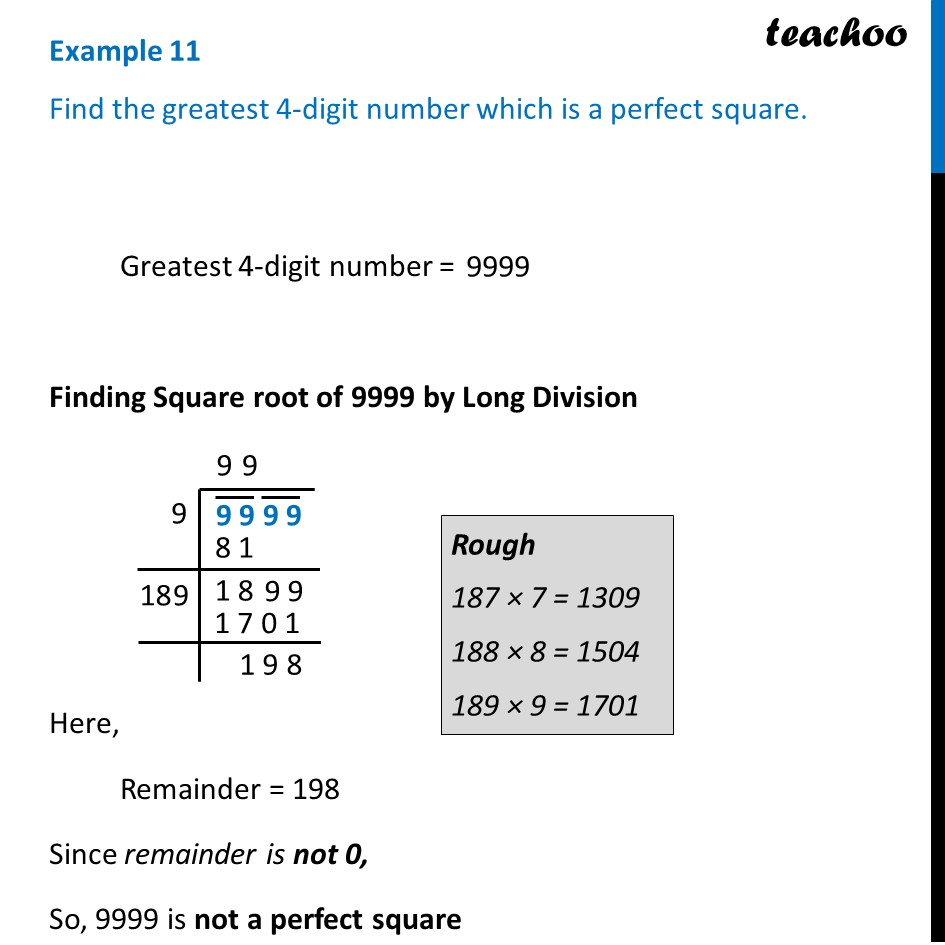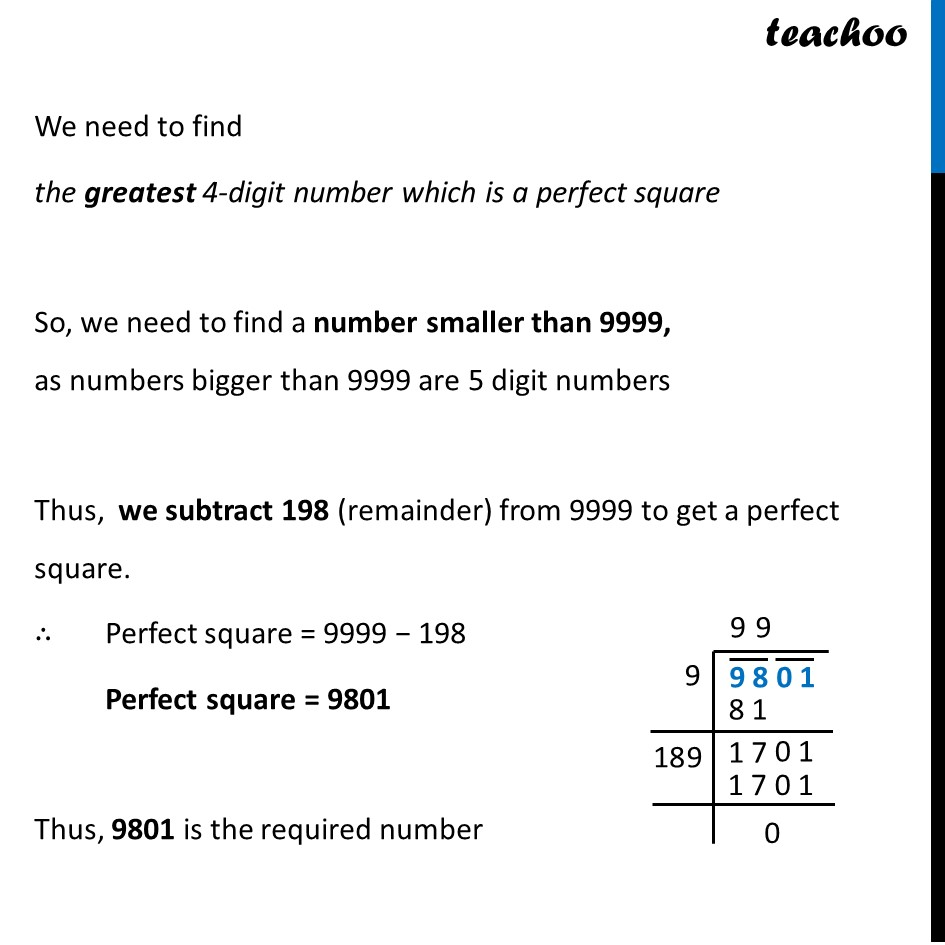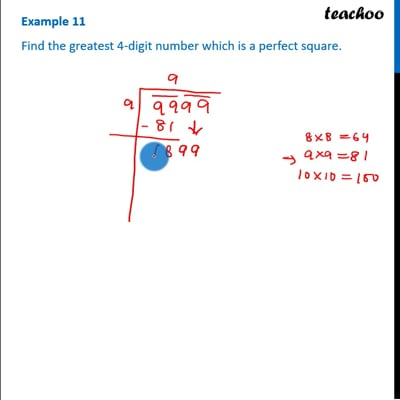Examples

Chapter 6 Class 8 Squares and Square Roots
Serial order wiseThis video is only available for Teachoo black users

### Transcript

Example 11 Find the greatest 4-digit number which is a perfect square. Greatest 4-digit number = Finding Square root of 9999 by Long Division Here, Remainder = 198 Since remainder is not 0, So, 9999 is not a perfect square We need to find the greatest 4-digit number which is a perfect square So, we need to find a number smaller than 9999, as numbers bigger than 9999 are 5 digit numbers Thus, we subtract 198 (remainder) from 9999 to get a perfect square. ∴ Perfect square = 9999 − 198 Perfect square = 9801 Thus, 9801 is the required number